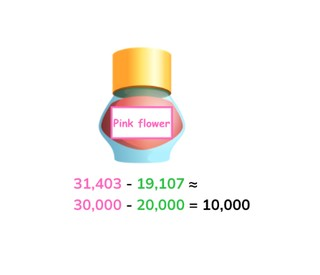Estimate the difference to 100,000

# Estimate the difference to 100,000

Learn to estimate the difference with numbers up to 100,000

No account needed.8,000 schools use Gynzy92,000 teachers use Gynzy1,600,000 students use Gynzy

## General

Students learn to estimate the difference with numbers up to 100,000 by rounding to the nearest thousand or ten thousands number.

## Standards

CCSS.Math.Content.4.NBT.B.4

## Learning objective

Students will be able to estimate the difference with numbers up to 100,000

## Introduction

Discuss how to round numbers and which number you look at to round. When rounding to a ten thousands number, you look at the number in the thousands place, and when rounding to a thousands number, you look at the number in the hundreds place. Repeat this using a number line as visual support. The interactive whiteboard shows six numbers. Ask the students to round these numbers and then draw a line to connect them with their rounded blue counterparts.

## Instruction

Explain to students that when estimating the difference you start by rounding the numbers in the problem to make an easier problem and then solving the rounded equation. The problem 49,183 - 10,722 ≈ can be solved using the estimated problem 50,000 - 11,000. Then use another example to explain that you can round the numbers to the nearest thousand or ten thousands number. Ask students to round each number and ask students to explain their strategy. Emphasize that, while estimating, there are different possible solutions. Practice matching the next three problems to their best estimated problems. Then, together with students, practice finding the estimated difference by rounding the numbers in the given problems and solving the estimated problem. Next practice estimating the difference and then solving for the exact difference. Together, discuss that the estimated difference and the exact difference are quite close to each other. Then explain that you can also estimate the difference with multiple numbers (more than 2). Remind students that they can also choose to add the multiple subtrahends together to subtract them together from the minuend. Practice with exercises in context of miles, where you ask about how many miles are left to fly.

Check that students are able to estimate difference with numbers to 100,000 by asking the following questions:
- Why is it useful to round numbers and then subtract using rounded numbers?
- When do you round a number to the nearest thousand or ten thousands number?
- How do you round numbers like 12,289 and 49,305?

## Quiz

Students are given ten questions in which they must determine which estimated problem matches the given problem, which problem matches the estimated problem given, round to the nearest thousand or ten thousands number, solve story problems, and solve estimated and exact differences.

## Closing

Discuss the importance of estimated difference with the class and remind them that it can help them quickly get close to the correct answer of a given problem. It can also help them to check their exact difference. Discuss when or why you would round to a nearest thousand or ten thousands number. To close, have them match the subtraction problems, the estimated difference and the differences together. As a last exercise, play a rounding game in which students must stand if they round up and sit if they round down.

## Teaching tips

Students who have difficulty with this learning goal can be supported by practicing with subtraction using ten thousands numbers. Make sure that students are not solving the exact problem and then rounding the difference that they find but that they start by rounding the numbers in the subtraction problem. Have them explain their rounding as they walk through solving the problem.

### The online teaching platform for interactive whiteboards and displays in schools

• Save time building lessons

• Manage the classroom more efficiently

• Increase student engagement